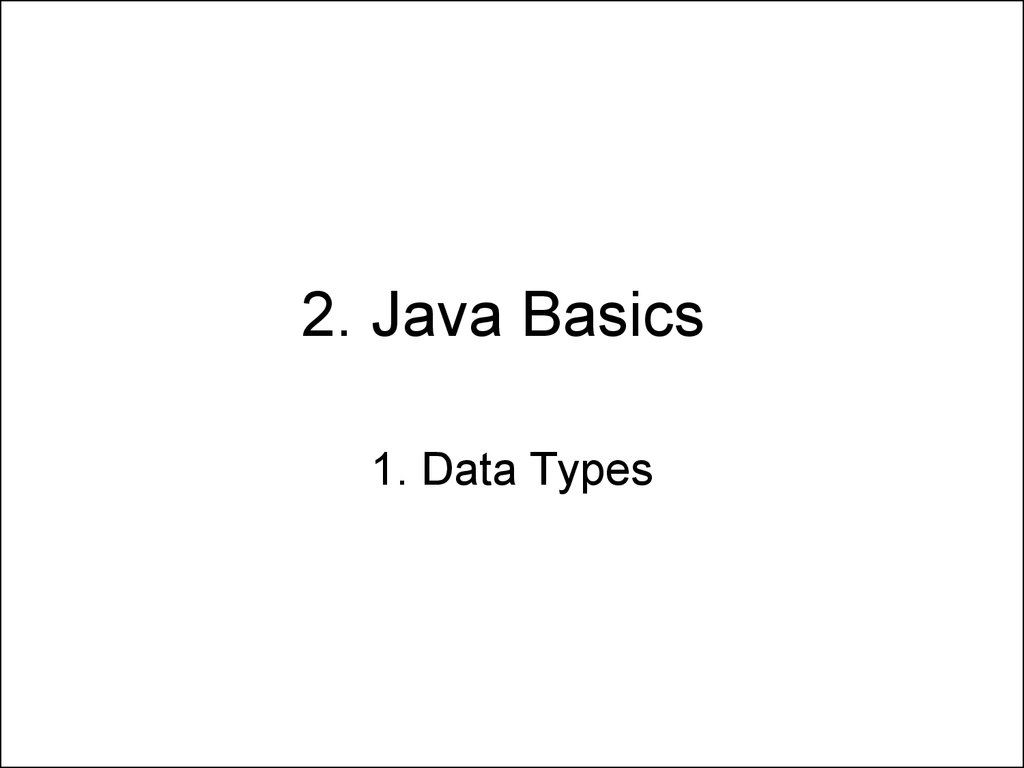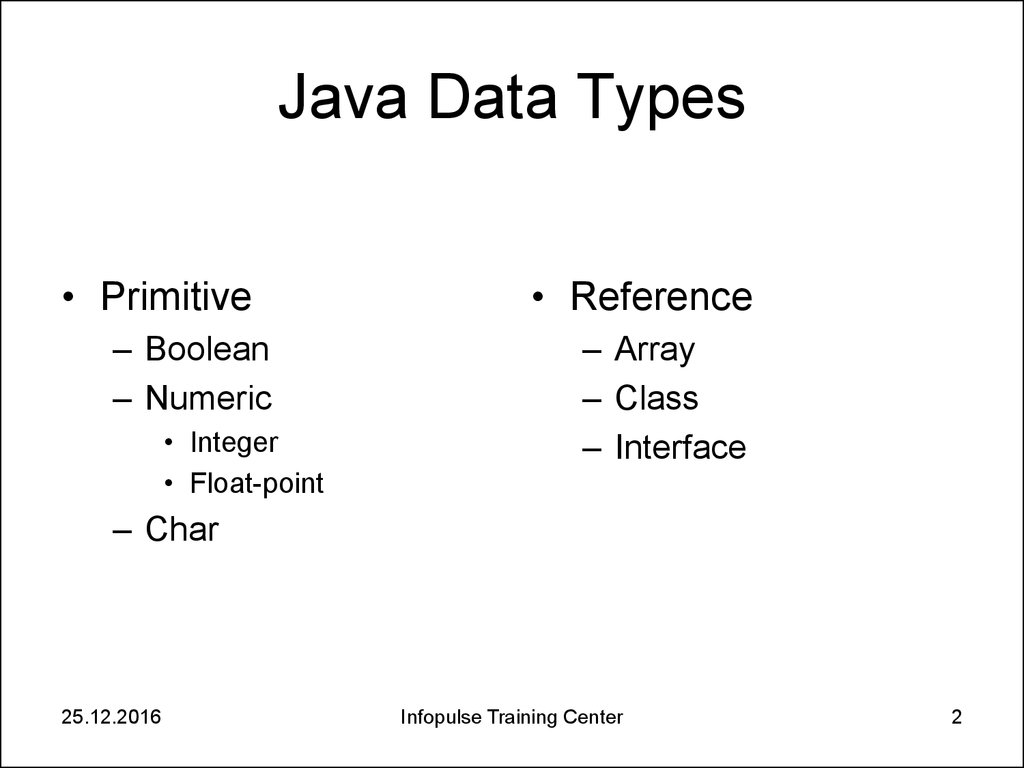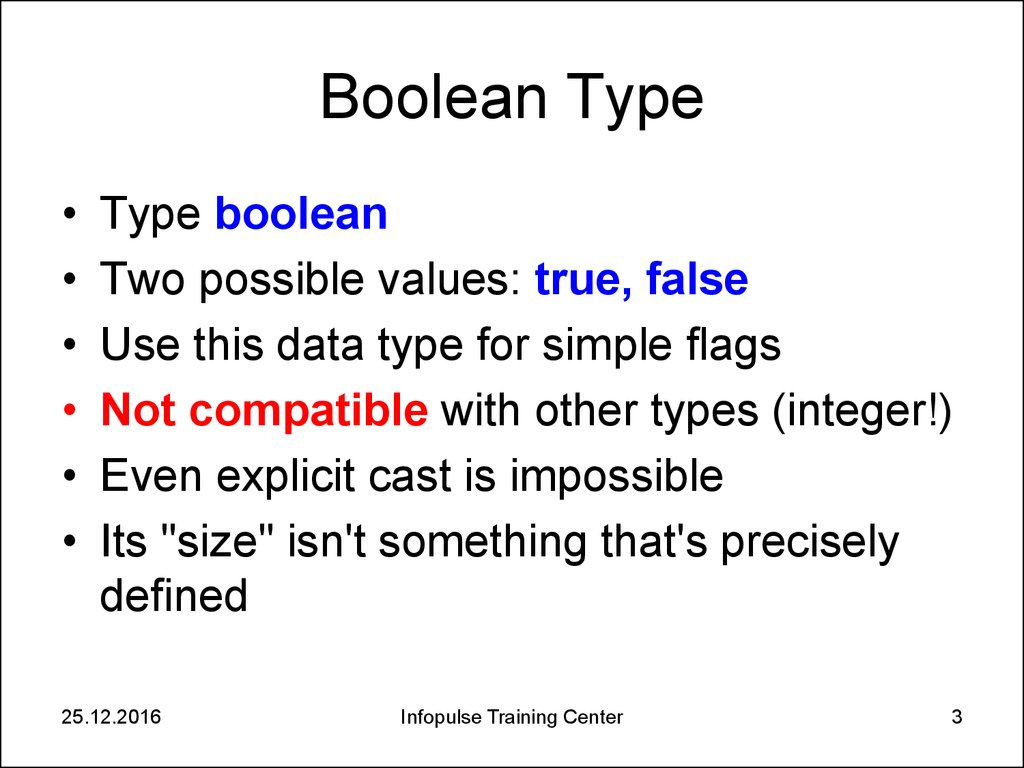# 2. Java Basics. Data Types

1. Data Types

## 2. Java Data Types

• Primitive
– Boolean
– Numeric
• Integer
• Float-point
• Reference
– Array
– Class
– Interface
– Char
25.12.2016
Infopulse Training Center
2

## 3. Boolean Type

Type boolean
Two possible values: true, false
Use this data type for simple flags
Not compatible with other types (integer!)
Even explicit cast is impossible
Its "size" isn't something that's precisely
defined
25.12.2016
Infopulse Training Center
3

## 4. Boolean Operators

=
== !=
!
&&
||
?:
&
|
25.12.2016
assignment
equal to, not equal to
NOT
AND
OR
if-then-else
bitwise AND
bitwise OR
Infopulse Training Center
4

## 5. If-Then-Else Boolean Operator

• expression1 ? expression2 : expression3
• Examples:
– BestReturn = Stocks > Bonds ? Stocks : Bonds;
– LowSales = JuneSales < JulySales ? JuneSales : JulySales;
– Distance = Site1 - Site2 > 0 ? Site1 - Site2 : Site2 - Site1;
25.12.2016
Infopulse Training Center
5

## 6. AND Boolean Operator

1. boolean a = false;
2. boolean b = true;
3. boolean c = a && b;
4. boolean d = a & b;
Will we get the same results for c and d?
25.12.2016
Infopulse Training Center
6

## 7. AND Boolean Operator

1. boolean a = false;
2. boolean b = true;
3. boolean c = a && b;
Operation && calculates first operand. If it
equals false, then returns false without
second operand calculation
4. boolean d = a & b;
Operation & calculates both operands and
then returns the result
25.12.2016
Infopulse Training Center
7

## 8. Integer Types

Type
Bytes
Min
Max
byte
1
-128
127
short
2
-32768
32767
int
4
-2 147 483 648
2 147 483 647
long
8
-9 223 372 036 854 775 808
9 223 372 036 854 775 807
All integer type are singed integer types
int is approximately in interval -2E9 to 2E9
long is approximately in interval -9E18 to 9E18
25.12.2016
Infopulse Training Center
8

## 9. Integer Literals

• Leading zero means octal constant (so 8 and 9
digits are impossible)
can use A-F or a-f as digits)
• Long constant ends with L or l symbols.
• Any number of underscore characters (_) can
appear anywhere between digits in a numerical
constants (since Java 7 only!)
25.12.2016
Infopulse Training Center
9

## 10. Integer Arithmetic Operations

+
*
/
%
25.12.2016
subtract
multiply
divide
get reminder
Infopulse Training Center
10

• byte a = 120;
• byte b = 10;
• byte c = (byte)(a + b);
What will be c value?
Why we use (byte)(a + b)?
25.12.2016
Infopulse Training Center
11

## 12. Integer Arithmetic Operations

• If one operand has long type then other
operand is converted to long. Otherwise
both operands are converted to int type.
• The result of an operation has int type if it
value does not need long type.
25.12.2016
Infopulse Training Center
12

## 13. Integer Assignment

• The integer assignment performs implicit
type conversion if neither accuracy nor
value is loss (e.g. int = byte or long = int)
• If implicit cast is impossible then explicit
cast is needed, otherwise compilation
error will occur ( e.g byte = (byte)int )
25.12.2016
Infopulse Training Center
13

## 14. Java Overflow And Underflow

• In Java arithmetic operators don’t report
overflow and underflow conditions
• When the result of an arithmetic integer
operation is larger than 32 bits then the
low 32 bits only taken into consideration
and the high order bits are discarded
• The same with long type (64 bits)
• It’s a shame of Java
25.12.2016
Infopulse Training Center
14

## 15. The Overflow Problem

• In Java arithmetic overflow will never
throw an exception
long a = 9223372036854775806L;
long b = 2L;
long c = a + b;
c = -9223372036854775808L
25.12.2016
Infopulse Training Center
15

## 16. Integer Division

x=a/b
r=a%b
int a = 20;
int b = 3;
int c = a / b;
int d = a % b;
What will be c and d values?
25.12.2016
Infopulse Training Center
16

## 17. Integer Division

Division by 0 leads to runtime ArithmeticException:
int a = 5;
int b = 0;
int c = a / b;
25.12.2016
Infopulse Training Center
17

## 18. The Integer Unary Operators

+
++
--
Unary plus operator
Unary minus operator
Increment operator
Decrement operator
• For pre-increment and pre-decrement (i.e., ++a
or --a), the operation is performed and the value
is produced.
• For post-increment and post-decrement (i.e.,
a++ or a--), the value is produced, then the
operation is performed.
25.12.2016
Infopulse Training Center
18

## 19. What will be a value?

• int x = 8;
• int a = x++ / x;
25.12.2016
Infopulse Training Center
19

## 20. What will be done?

• int c = 10;
• int d = c+++++c;
25.12.2016
Infopulse Training Center
20

## 21. What will be done?

int c = 10;
int d = c++ + ++c;
25.12.2016
Infopulse Training Center
21

## 22. Bitwise Operators

~
&
|
^
25.12.2016
inverts a bit
bitwise AND
bitwise OR
bitwise inclusive OR
Infopulse Training Center
22

## 23. Bitwise Operators

int a = 45;
int b = 34;
int c = a ^ b;
What will be c value?
int d = c ^ b;
What will be d value?
25.12.2016
Infopulse Training Center
23

## 24. Bit Shift Operators

• <<
• >>
• >>>
25.12.2016
signed left shift operator
signed right shift operator
right shift operator
Infopulse Training Center
24

## 25. Bit Shift Operators

int a = 45;
int b = a >> 3;
b=?
int c = a << 3;
c=?
25.12.2016
Infopulse Training Center
25

## 26. Integer Assignment Operators

=
+=, -=, *=, /=
<<=, >>=, >>>=
&=, |=, ^=
25.12.2016
Infopulse Training Center
26

## 27. Integer Assignment Operators

• x += 1; instead x = x + 1;
• a *= 5; instead a = a * 5;
25.12.2016
Infopulse Training Center
27

## 28. The Equality and Relational Operators

==
!=
>
>=
<
<=
25.12.2016
equal to
not equal to
greater than
greater than or equal to
less than
less than or equal to
Infopulse Training Center
28

## 29. Float point Data Types

• float – 32 bit
(± 1E38, 7-8 dec. precision)
• double – 64 bit (± 1E308, 16-17 dec. precision)
Accordingly IEEE 754-1985 standard
25.12.2016
Infopulse Training Center
29

## 30. Float point Arithmetic Operations

+
*
/
25.12.2016
subtract
multiply
divide
Infopulse Training Center
30

## 31. Float point Arithmetic Operations

• If one operand has double type then other
operand is converted to double and result
will be double type.
• If one operand has float type and other
operand has any type differs from double
then other operand is converted to float
and result will be float type
25.12.2016
Infopulse Training Center
31

## 32. What will be c and d value?

double a = 2.2;
double b = -1.4;
a = a - 2.2;
double c = b / a;
double d = Math.sqrt(b);
25.12.2016
Infopulse Training Center
32

## 33. Special Float Point Values

• -Infinity
• +Infinity
• NaN
In previous code c = -Infinity, d = NaN
25.12.2016
Infopulse Training Center
33

## 34. Precision Problem I

• double a = 2.0;
• double b = a - 1.1;
b will be 0.8999999999999999, not 0.9!
25.12.2016
Infopulse Training Center
34

## 35. Precision Problem II

How many repetitions will be?
double d = 0.1;
while (d != 1.0) {
System.out.println(d);
d += 0.1;
}
25.12.2016
Infopulse Training Center
35

## 36. Debugging in Eclipse

• Start debugging: press Debug icon and use
F6 key for stepped debugging
• Use Cntr + Shift + B for breakpoint creation
• Use Cntr + R to run application to the next
breakpoint
25.12.2016
Infopulse Training Center
36

## 37. Precision Problem Source

Above precision problems caused by the
fact that finite decimal fraction 0.1 is infinite
periodical binary fraction:
1
1 1 1 1
( )
10 16 32 16 10
So 0.1 can be represented as binary fraction
in a computer only approximately.
25.12.2016
Infopulse Training Center
37

## 38. Float point Literals

Here are possible formats for
float point constants
Suffix f(F) means
• 1003.45
float constant,
suffix d(D) –
• .00100345e6
double constant.
• 100.345E+1
Constant without
• 100345e-2
suffix - double
• 1.00345e3
• 0.00100345e+6
25.12.2016
Infopulse Training Center
38

## 39. The Float point Unary Operators

+
++
--
25.12.2016
Unary plus operator
Unary minus operator
Increment operator
Decrement operator
Infopulse Training Center
39

## 40. Float point Assignment Operators

• =
• +=, -=, *=, /=
25.12.2016
Infopulse Training Center
40

## 41. The Equality and Relational Operators

==
!=
>
>=
<
<=
25.12.2016
equal to
not equal to
greater than
greater than or equal to
less than
less than or equal to
Infopulse Training Center
41

## 42. Char Type

• The char data type is a single 16-bit
Unicode character
• Char data can be processed as unsigned
short integers (0 – 65535) too.
25.12.2016
Infopulse Training Center
42

## 43. Char Literals

• A symbol: 'a', 'A', '9', '+', '_', '~' (except \)
• Unicode symbol: '\u0108'
• Escape sequences '\b' '\t' '\n' '\f' '\r' '\"' '\'' '\\'
Don’t confuse char and string literals (e.g. ‘r’ and “r”)!
The \uxxxx notation can be used anywhere in the source to
represent unicode characters
25.12.2016
Infopulse Training Center
43

## 44. Char Examples

char c = 'g';
System.out.println(++c);
char r = (char)(c ^ 32);
25.12.2016
Infopulse Training Center
44

## 45. Expressions. Operator precedence

. [] ()
+ - ~ ! ++
* / %
+ << >> >>>
< <= >= >
== !=
25.12.2016
--
instanceof
Infopulse Training Center
&
^
|
&&
||
?:
= op=
45

## 46. Casting (1 of 2)

• Any integer type can be casted to any
other primitive type except boolean
• Casting from larger integer type to smaller
(from long to short for example) can lead
to data loss
• Casting from integer type to float point
type can lead to precision loss (if integer is
not power of 2)
25.12.2016
Infopulse Training Center
46

## 47. Casting (2 of 2)

• Char type casting is the same as short
integer type casting.
• Casting from float or double types to
integer types returns integer part of the
value without rounding
25.12.2016
Infopulse Training Center
47

## 48. Casting operators (1 of 2)

• Implicit casting:
byte b = 18;
int a = b;
• Explicit casting:
int a = 18;
byte b = (byte)a;
25.12.2016
Infopulse Training Center
48

## 49. Casting operators (2 of 2)

• int b = 168;
double a = b;
• float p = 18.94f;
byte b = (byte)p; // b = 18
25.12.2016
Infopulse Training Center
49

## 50. Manuals

• Learning the Java Language. Language
Basics
• Thinking in Java. Operators.
25.12.2016
Infopulse Training Center
50